• +91 9971497814
• info@interviewmaterial.com

# Chapter 2- Solutions Interview Questions Answers

### Related Subjects

Question 1 :

Calculate the mass percentage ofbenzene (C6H6) and carbon tetrachloride (CCl4) if 22 g of benzene is dissolved in 122 g ofcarbon tetrachloride.

Answer 1 :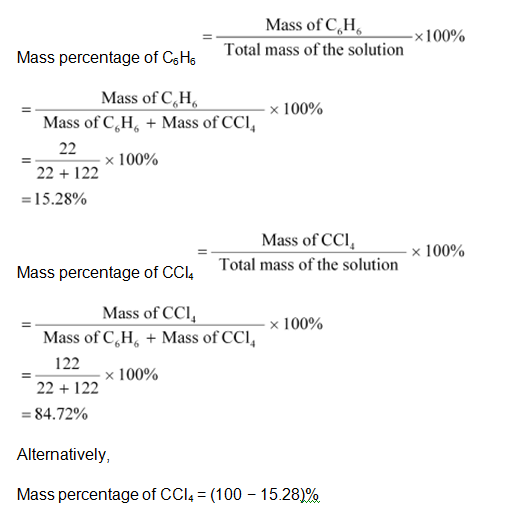= 84.72%

Question 2 :

Calculate the mole fraction ofbenzene in solution containing 30% by mass in carbon tetrachloride.

Let the total mass of thesolution be 100 g and the mass of benzene be 30 g.

Mass ofcarbon tetrachloride = (100 − 30)g

= 70 g

Molar mass of benzene (C6H6) = (6 × 12 + 6 × 1) g mol−1

= 78 g mol−1

Numberof moles of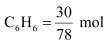= 0.3846 mol

Molar mass of carbon tetrachloride (CCl4) = 1 × 12 + 4 × 35.5

= 154 g mol−1

Numberof moles of CCl4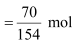= 0.4545 mol

Thus, the molefraction of C6H6 is given as: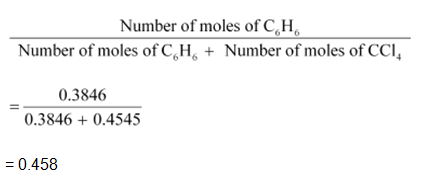Question 3 :

Calculate the molarity of each ofthe following solutions: (a) 30g of Co(NO3)2. 6H2O in 4.3 L of solution (b) 30 mL of 0.5 M H2SO4 diluted to 500 mL.

Molarity is given by: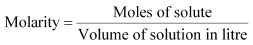a) Molar mass of Co (NO3)2.6H2O = 59 + 2 (14 + 3 × 16) + 6 × 18

= 291 g mol−1

Molesof Co (NO3)2.6H2O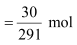= 0.103 mol

Therefore, molarity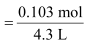= 0.023 M

(b) Number of moles present in 1000 mL of 0.5 M H2SO4 = 0.5 mol

Numberof moles present in 30 mL of 0.5 M H2SO4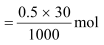= 0.015 mol

Therefore, molarity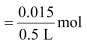= 0.03 M

Question 4 :

Calculate the mass of urea (NH2CONH2) required in making 2.5 kg of 0.25 molalaqueous solution.

Molar mass of urea (NH2CONH2) = 2(1 × 14 + 2 × 1) + 1 × 12 + 1 × 16

= 60 g mol−1

0.25 molar aqueous solution of urea means:

1000 g ofwater contains 0.25 mol = (0.25 × 60)g of urea

= 15 g ofurea

That is,

(1000 + 15) g of solution contains 15 g of urea

Therefore,2.5 kg (2500 g) of solution contains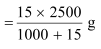= 36.95 g

= 37 g of urea (approximately)

Hence, mass of urea required = 37g

Question 5 :

Calculate (a) molality (b) molarityand (c) mole fraction of KI if the density of 20% (mass/mass)aqueous KI is 1.202 g mL-1.

(a) Molarmass of KI = 39 + 127 = 166 g mol−1

20% (mass/mass)aqueous solution of KI means 20 g of KI is present in 100 g of solution.

That is,

20 g of KI is present in (100 −20) g of water = 80 g of water

Therefore,molality of the solution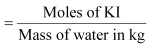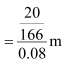= 1.506 m

= 1.51 m (approximately)

(b) Itis given that the density of the solution = 1.202 g mL−1

Volume of 100 gsolution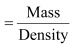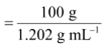= 83.19 mL

= 83.19 × 10−3 L

Therefore,molarity of the solution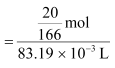= 1.45 M

(c) Molesof KI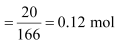Moles of water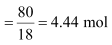Therefore, mole fraction of KI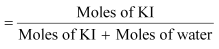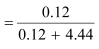= 0.0263

Question 6 :

H2S, a toxic gas withrotten egg like smell, is used for the qualitative analysis. If the solubilityof H2S in water at STP is 0.195 m, calculate Henry’s law constant.

It is given that thesolubility of H2S in water at STP is 0.195 m, i.e., 0.195 mol of H2Sis dissolved in 1000 g of water.

Molesof water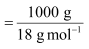= 55.56 mol

Mole fraction of H2S, x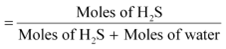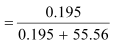= 0.0035

At STP, pressure (p) =0.987 bar

According to Henry’s law:

p = KHx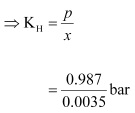= 282 bar

Question 7 :

Henry’s law constant for CO2 inwater is 1.67 × 108 Pa at 298 K. Calculate the quantity of CO2 in500 mL of soda water when packed under 2.5 atm CO2 pressure at298 K.

It is given that:

KH = 1.67 × 108 Pa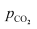= 2.5 atm = 2.5 × 1.01325 ×105 Pa

= 2.533125 × 105 Pa

According to Henry’s law: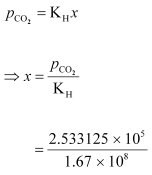= 0.00152

We can write,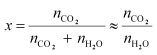[Since,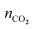is negligible as compared to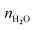]

In 500 mL of soda water, thevolume of water = 500 mL

[Neglecting the amount of sodapresent]

We can write:

500 mL of water = 500 g of water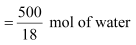= 27.78 mol of water

Now,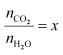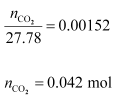Hence, quantity of CO2 in500 mL of soda water = (0.042 × 44)g

= 1.848 g

Question 8 :

The vapour pressure of pureliquids A and B are 450 and 700 mm Hg respectively, at 350 K. Find out thecomposition of the liquid mixture if total vapour pressure is 600 mm Hg. Alsofind the composition of the vapour phase.

Answer 8 :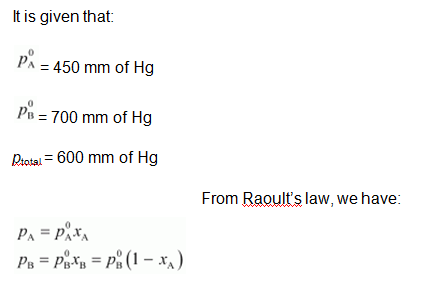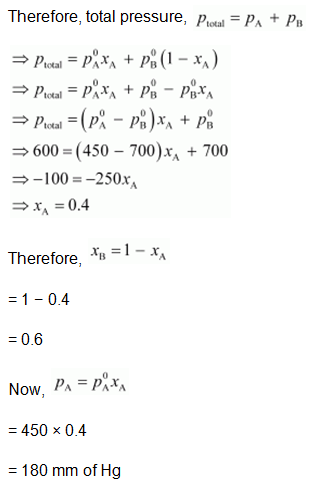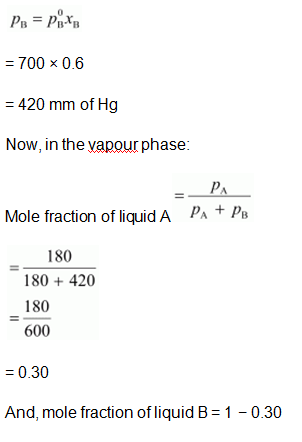= 0.70

Question 9 :

Vapour pressure of pure water at298 K is 23.8 mm Hg. 50 g of urea (NH2CONH2) is dissolvedin 850 g of water. Calculate the vapour pressure of water for this solution andits relative lowering.

Answer 9 :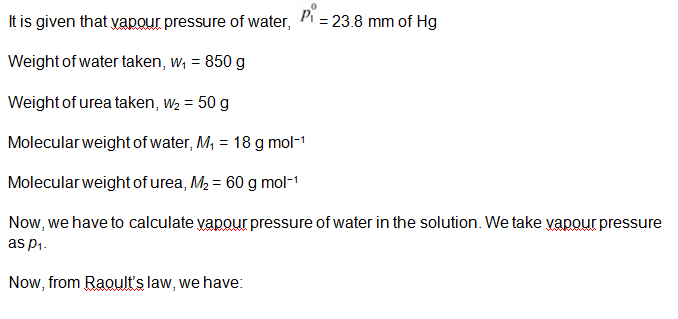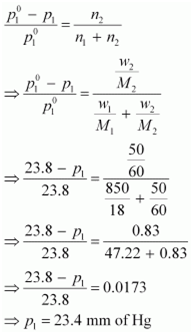Hence, the vapour pressure ofwater in the given solution is 23.4 mm of Hg and its relative lowering is0.0173.

Question 10 :

Boiling point of water at 750 mmHg is 99.63°C. How much sucrose is to be added to 500 g of water such that itboils at 100°C. Molal elevation constant for water is 0.52 K kg mol−1.

Here, elevation of boiling pointΔTb = (100 + 273) − (99.63 + 273)

= 0.37 K

Mass of water, wl =500 g

Molar mass of sucrose (C12H22O11), M2 =11 × 12 + 22 × 1 + 11 × 16

= 342 g mol−1

Molal elevation constant, Kb =0.52 K kg mol−1

We know that: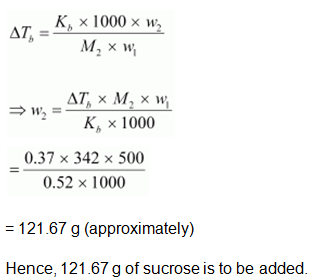Todays Deals### Chapter 2- Solutions Contributorskrishan

Name:
Email:

# Latest News# 9000 interview questions in different categories